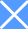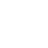发送至邮箱

﻿
nn
n
n

(略) 省 (略) 会保障厅 (略) 上超市采购项目（项目编号: *** ）采购已经结束，现将采购结果公示如下：

n
n

* 、项目信息

n

n

n

n

n

n

n

n

(略) 在行政区划编码: ***

n

(略) 在行政区划名称: (略) 省本级

n

n

* 、采购单位信息

n

n

n

n

(略) 会统 * 信用代码或组织机构代码: *** G

n

n

* 、成交信息

n

n

n

n

n

nn n n n nnn n n n nn
 序号 成交供应商名称 成交供应商地址 成交金额（元） 1 (略) (略) 省 (略) 市 (略) 省 (略) 市 (略) 区 (略) 街道 (略) 东路 * 号 (略) 电子世界 * 3房 * .0

n

* 、项目用途、简要技术要 (略) 日期:

n n

* 、成交标的名称、规格型号、数量、单价、成交金额:

n

n

nn n n n n n n n nnn n n n n n n n nnn n n n n n n n nnn n n n n n n n nnn n n n n n n n nnn n n n n n n n nnn n n n n n n n nn
 序号 标的名称 品牌 规格型号 数量 单价(元) 成交金额（元） 报价明细 1 惠普/HP * 黑/红/青/黄 标准容量墨盒 适用 * * * * * * 惠普/HP * 4 * .0 * .0 2 惠普/HP * 黑/红/青/黄 标准容量墨盒 适用 * * * * * * 惠普/HP * 2 * .0 * .0 3 惠普/HP * 黑/红/青/黄 标准容量墨盒 适用 * * * * * * 惠普/HP * 2 * .0 * .0 4 惠普/HP * 黑/红/青/黄 标准容量墨盒 适用 * * * * * * 惠普/HP * 2 * .0 * .0 5 (略) 楼复印纸 * K * G 8包/箱复印纸打印纸 (略) 楼 * K * G 8包 2 * .0 * .0 6 【运费】 1 0.0 0.0

n

* 、保证金金额、 (略) 、用户名及卡号:

n n

* 、其他补充事宜:

n n
n
n
﻿
nn
n
n

(略) 省 (略) 会保障厅 (略) 上超市采购项目（项目编号: *** ）采购已经结束，现将采购结果公示如下：

n
n

* 、项目信息

n

n

n

n

n

n

n

n

(略) 在行政区划编码: ***

n

(略) 在行政区划名称: (略) 省本级

n

n

* 、采购单位信息

n

n

n

n

(略) 会统 * 信用代码或组织机构代码: *** G

n

n

* 、成交信息

n

n

n

n

n

nn n n n nnn n n n nn
 序号 成交供应商名称 成交供应商地址 成交金额（元） 1 (略) (略) 省 (略) 市 (略) 省 (略) 市 (略) 区 (略) 街道 (略) 东路 * 号 (略) 电子世界 * 3房 * .0

n

* 、项目用途、简要技术要 (略) 日期:

n n

* 、成交标的名称、规格型号、数量、单价、成交金额:

n

n

nn n n n n n n n nnn n n n n n n n nnn n n n n n n n nnn n n n n n n n nnn n n n n n n n nnn n n n n n n n nnn n n n n n n n nn
 序号 标的名称 品牌 规格型号 数量 单价(元) 成交金额（元） 报价明细 1 惠普/HP * 黑/红/青/黄 标准容量墨盒 适用 * * * * * * 惠普/HP * 4 * .0 * .0 2 惠普/HP * 黑/红/青/黄 标准容量墨盒 适用 * * * * * * 惠普/HP * 2 * .0 * .0 3 惠普/HP * 黑/红/青/黄 标准容量墨盒 适用 * * * * * * 惠普/HP * 2 * .0 * .0 4 惠普/HP * 黑/红/青/黄 标准容量墨盒 适用 * * * * * * 惠普/HP * 2 * .0 * .0 5 (略) 楼复印纸 * K * G 8包/箱复印纸打印纸 (略) 楼 * K * G 8包 2 * .0 * .0 6 【运费】 1 0.0 0.0

n

* 、保证金金额、 (略) 、用户名及卡号:

n n

* 、其他补充事宜:

n n
n
n

 招标 代理 - 关注我们可获得更多采购需求  已关注

 查看详情  收藏  首页